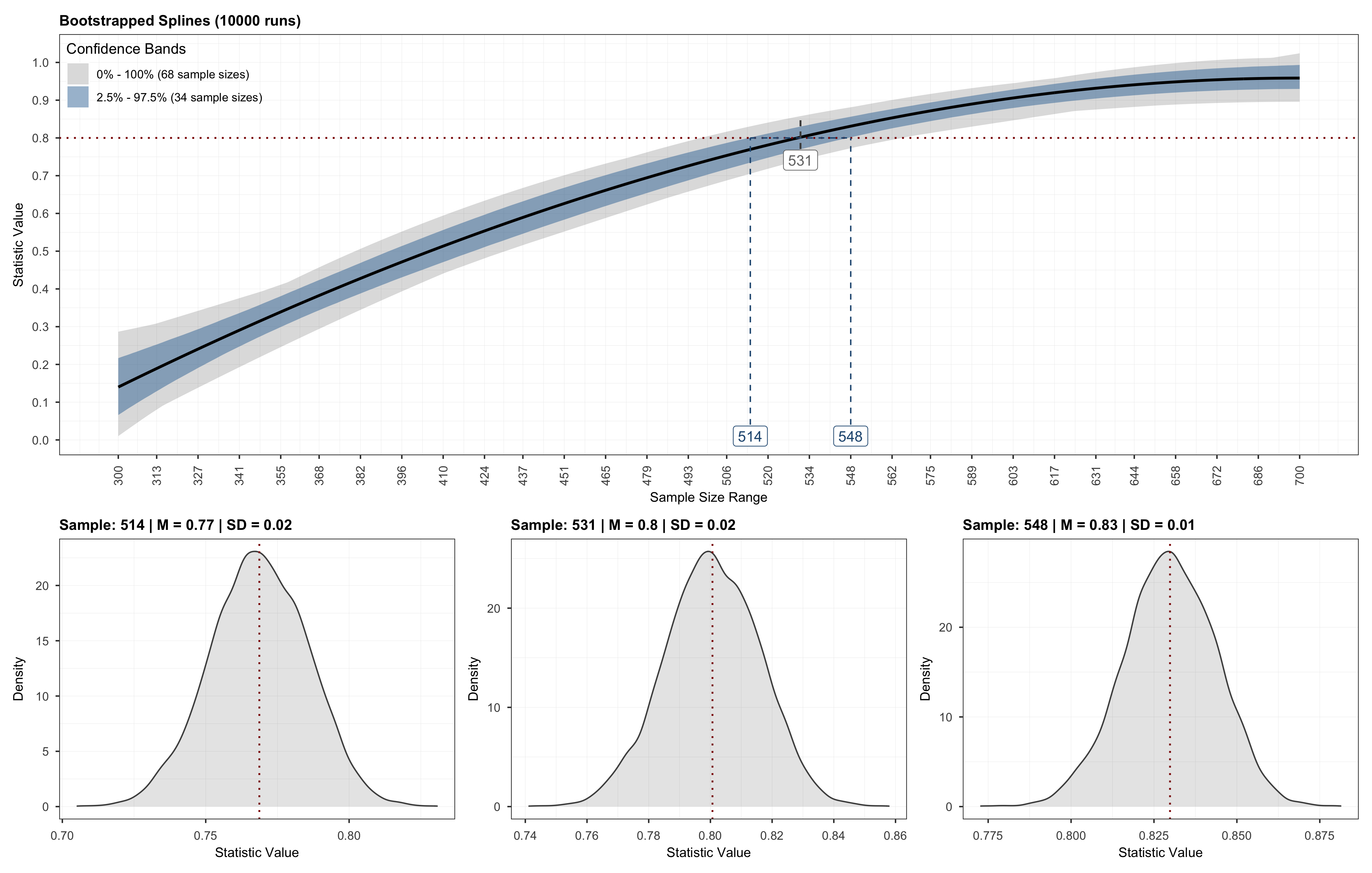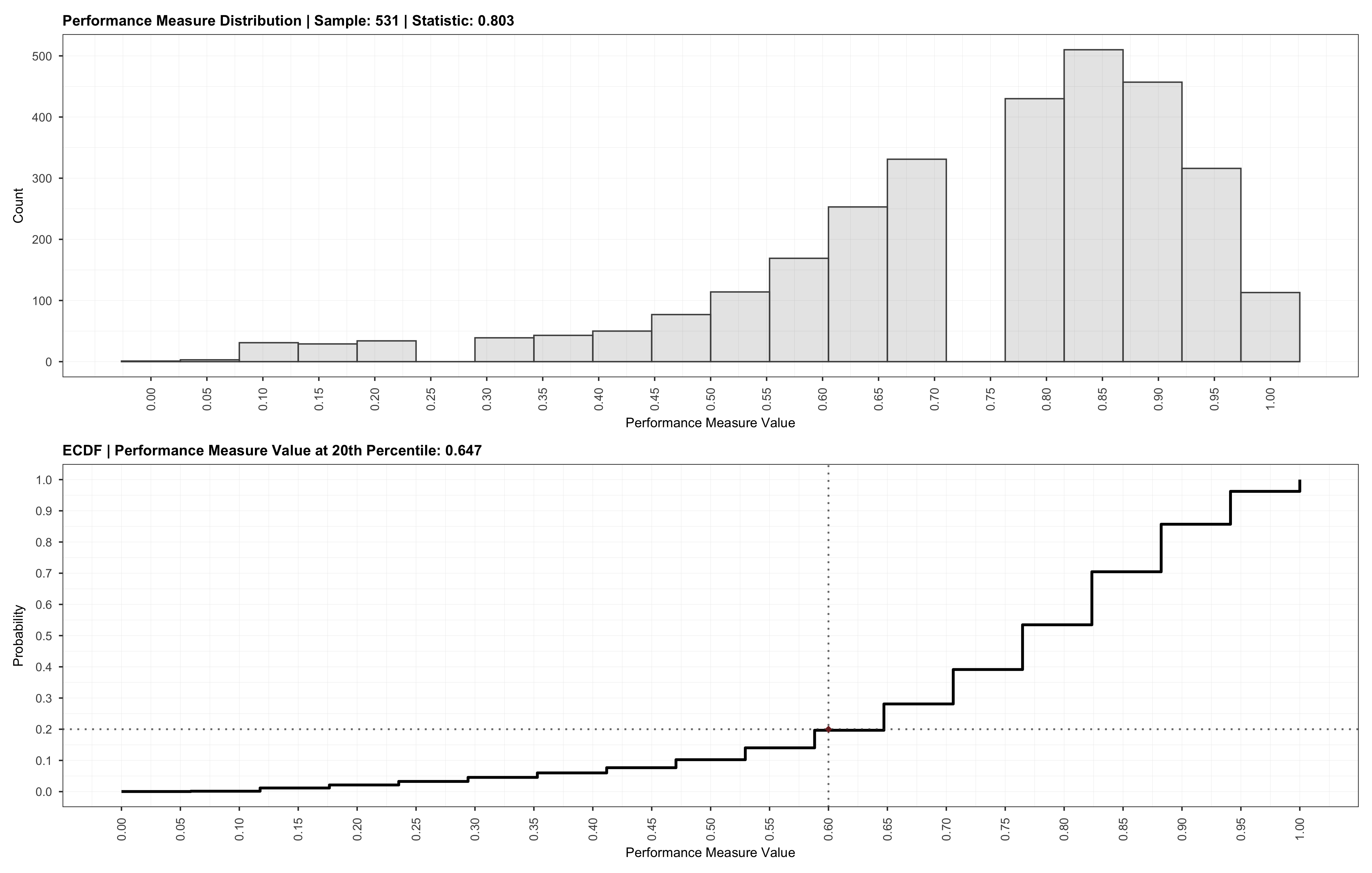# # Introduction

powerly is an R package developed to provide support for researchers who wish to perform power analysis. At its core, powerly is based on the method proposed by Constantin et al. (2021)open in new window, which takes the form of a three-step algorithm designed to iteratively search for an optimal sample size, given some user input. It takes as input a set of hypothesized true model parameters (i.e., an effect size), a performance measure of interest and its corresponding target value (e.g., a sensitivity of ), and a statistic that describes how the performance measure value should be reached (e.g., reaching a sensitivity of with a probability of ). The search for the optimal sample size is conducted in three steps, starting with a Monte Carlo simulation step for computing the performance measure and statistic at various sample sizes within a candidate sample size range. It continues with a monotone curve-fitting step for interpolating the statistic. The final step employs stratified bootstrapping to quantify the uncertainty around the fitted curve. For more details, consult the manuscriptopen in new window or check out the remainder of the Tutorial Section.

Next, I will provide a quick overview on how to get started with powerly, list the main functions available in the package, and highlight the main steps involved in using powerly for running a power analysis.

TIP

If you are interested in building upon or extending powerly, check out the Developer Section where the package API and the design choices are discussed in detail.

## # Installation

You can install the latest version of powerly from CRANopen in new window (i.e., the official R package repository) running the following command:

install.packages("powerly")


Alternatively, you can install the latest development versionopen in new window from GitHub via:

Click to expand optional step.
# Install the remotes package if necessary.
install.packages("remotes")

remotes::install_github("mihaiconstantin/powerly")


TIP

You may consult the Newsopen in new window or the GitHub releasesopen in new window for an overview of the latest changes in the development version.

## # Functions

powerly exports three functions available to researchers for conducting sample size analysis.

Function NameDescription
generate_modelUsed for generating true models parameters when manually specifying them is non-trivial.
powerlyThe main function of the package implementing the sample size calculation method described above.
validateUsed for assessing the quality of the sample size recommendation obtained after running the powerly function.

TIP

Check out the Reference Section corresponding to each function for detailed information about the available arguments and the output provided.

## # Usage

We start by attaching the powerly package.

# Load the package.
library(powerly)


Suppose we want to find the sample size required to observe a sensitivity of with a probability of for an arbitrary Gaussian Graphical Model (GGM) consisting of nodes with an edge density of .

We start by specifying the true model (i.e., in this case an edge weights matrix encoding of partial correlation coefficients), or we can generate one using the generate_model function. For example, this can be achieved by running:

Click to see the seed used for reproducibility.

We may set a seed to ensure that we generate the same true model. While this will not lead to the exact same sample size recommendation for each repeated run of the method (i.e., due to the randomness involved in the Monte Carlo procedure), the method will still, on average, provide the optimal sample size value (i.e., around for this particular example).

# Set a seed.
set.seed(20031993)

# Generate a GGM model.
true_model <- generate_model(
type = "ggm",
nodes = 10,
density = .4
)


Then, we can supply the true_model object to the powerly function to start the search for the sample size. Alongside the true_model we may also specify a number of other arguments that constitute the method parameters.

TIP

You may also run ?powerly in R to bring up the documentation and learn more about the role of each argument in the powerly function.

# Run the method.
results <- powerly(
range_lower = 300,
range_upper = 700,
samples = 30,
replications = 40,
measure = "sen",
statistic = "power",
measure_value = .6,
statistic_value = .8,
model = "ggm",
model_matrix = true_model,
cores = 7
verbose = TRUE
)


At this point we can print the results.

Method run completed (2.943 sec):
- converged: yes
- iterations: 1
- recommendation: 531


Or better visualize them.

# Plot method run.
plot(results)


Which will produce a plot similar to the one below.Optionally, we may also validate the sample size recommendation using the validate function by passing in the output obtained from the powerly function, followed by plotting the validation results.

# Run validation.
validation <- validate(
method = results,
replications = 3000,
cores = 9
)

# Plot validation.
plot(validation)


Which will result in the following:## # Next Steps

This introduction is intended to provide the bare minimum information needed to get started with powerly. If you want to learn more about where the numbers come from, I encourage you to check out the manuscriptopen in new window, or read the next post where I succinctly describe the terminology and the steps of the method. From there, you may continue with applications of the methods to different models (e.g., psychological networks, structural equation models etc.), or by checking out the posts under the FAQ, where I discuss tips and tricks for how to efficiently use powerly.